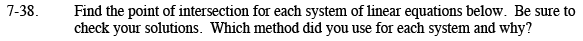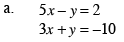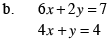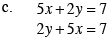### Home > CAAC > Chapter 7 > Lesson 7.1.4 > Problem7-38

7-38.Use the Elimination Method.

Add the equations together vertically.
The result is: 8x = −8

Solve for x by dividing the equation by 8. x = −1

Substitute x into one of the original equations to find y. 5(−1) − y = 2

Solve for y.
y = −7

(−1, −7)Use the Elimination Method.

Multiply the 2nd equation by −2

Follow the steps outlined in part (a).Try rewriting the 2nd equation. What do you notice?